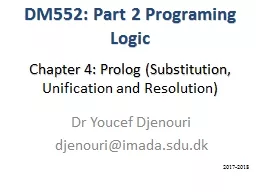# Chapter 4: Prolog (Substitution,2018-03-19 18K 18 0 0

## Chapter 4: Prolog (Substitution, - Description

Unific. ation. . and . Resolution. ). Dr Youcef Djenouri. djenouri@imada.sdu.dk. D. M552: Part . 2 . Programing. . Logic. 2017-2018. Substitution: . Definition. (1/2). Substitution. . θ . is . an operation allowing . ID: 656674 Download Presentation

Embed code:

## Chapter 4: Prolog (Substitution,

Download Presentation - The PPT/PDF document "Chapter 4: Prolog (Substitution," is the property of its rightful owner. Permission is granted to download and print the materials on this web site for personal, non-commercial use only, and to display it on your personal computer provided you do not modify the materials and that you retain all copyright notices contained in the materials. By downloading content from our website, you accept the terms of this agreement.

### Presentations text content in Chapter 4: Prolog (Substitution,

Slide1

Chapter 4: Prolog (Substitution, Unification and Resolution)

DM552: Part 2 Programing Logic

2017-2018

Slide2

Substitution: Definition (1/2)Substitution θ is an operation allowing to replace some variables occurring in a formula with terms.

The goal of applying a substitution is to make a certain formula more specific so that it matches another formula. Substitutions allow for unification of formulae (or

terms).

Slide3

Substitution: Definition (2/2)A substitution is any finite mapping of variables into terms of the form: θ

: V ⇒ TERAny (ﬁnite) substitution θ can be presented asθ={t

1/X1, t2/X2… tn/Xn}ti is the term to be substituted for a variable Xi, i= 1…n. No two elements in the set have the same variable after the / symbol,

Slide4

Substitution: Example{ y/X, f(y)/Z } is a substitution. { a/Y, b/X}

is a substitution.{a/X, b/X} is not a substitution.{X/f(Y)}

is not a substitution.

Slide5

Instance (1/2) Let θ ={t1/X1, t2/X2…

tn/Xn} be a substitution and let E be an expression. Then E

θ is an expression obtained by replacing all occurrences of every vi in E with the corresponding term ti, Eθ is an instance of E.

Slide6

Instance (2/2) θ ={ a/X, f(b)/Y, c/Z} , E=P(X, Y, Z)

Eθ= P(a, f(b), c) is an instance of E. θ ={f(f(a))/X, X/Y} , E

=(P(X ) ∨ Q(Y)) Eθ= (P(f(f(a))) ∨ Q(X)) is an instance of E. θ ={ Y/X, a/Y} , E=(P(X ) ∨ Q(Y)) Eθ= (P(Y) ∨ Q(a)) is an instance of E.

Slide7

Composition of substitutionsLet θ= {t1/X1, t2/X2…

tn/Xn} and Γ={

u1/Y1, u2/Y2… um/Ym} be two substitutions. The composition of θ and Γ is denoted by θ o Γ, and it is obtained by building the set {t1 Γ

/

X

1

,

t

2

Γ

/

X

2

t

n

Γ

/

X

n

,

u

1

/Y

1

,

u

2

/Y

2

u

m

/

Y

m

}

R

emove

the

following

elements

:

t

j

Γ

/

X

j

such that

t

j

Γ

=

X

j

u

i

/

Y

j

such that

Y

j

is in {X

1

,

X

2

X

n

}

Slide8

Exampleθ= {t1/X1, t2/X2}= {

f(Y)/X, Z/Y} Γ={u1

/Y1, u2/Y2,u3/Y3}= {a/X, b/Y, Y/Z}{t1 Γ /X1, t2 Γ /X2, u1/Y1, u2/Y2,u3/Y3

}={f(b

)/X,

Y

/Y

,

a/X

,

b/Y

,

Y

/Z}

θ

o

Γ

={f(b

)/X,

Y

/Z}

Slide9

ClassworkConsider θ = {f(Y)/X} and µ = {

f(Z)/Y} Compute θ o µ and µ o

θ ?

Slide10

UnificationA substitution θ is called a unifier for a set {E1…Ek} if and

only if: E1θ =E2θ = E

3θ =…= EkθThe set {E1…Ek} is unifiable if and only if there exists a unifier for it. A unifier θ is a most general unifier for a set {E1…Ek} if and only if for each

unifier

Γ

there

exisits

a substitution

μ

such

that

Γ

= θ o

μ

.

Slide11

ExampleE1=P(X), E2= P(f(Y)) are unifiable by

Γ={f(f(Z))/X, f(Z)/Y}E

1 Γ = E2 Γ = P(f(f(Z))). θ= {f(Y)/X} is a most general unifier, we can find μ ={f(Z)/Y} such that θ o μ= {f(f(Z))/X, f(Z)/

Y

}=

Γ

Slide12

ClassworkProve that the following expressions are all unifiers by θ = {f(b)/X, b/Y, u/Z}

E = f(X, b, g(Z)).

F = f(f(Y), Y, g(u)).

Slide13

Resolution: Used to prove a consequence from a set of logical formulaeStates: Sets of clauses L1

…  LmInitially: The consequence we want to prove is negated and added to the set of clauses representing the initial state

Goal: Derive the empty clause ()

Slide14

Example (1/6)¬P(X)  P(f(X))

¬Q(a, Y)  ¬R(Y, X

)  P(X)R(b, g(a, Z))Q(a, b)Goal: P(f(g(a, c)))

Slide15

Example (2/6)Negate goal, add to formulas1. ¬P(X) 

P(f(X))2. ¬Q(a, Y)  ¬R(Y, X)

 P(X)3. R(b, g(a, Z))4. Q(a, b)5. ¬P(f(g(a, c)))

Slide16

Example (3/6)Resolve (4) and (2) by applying {b/Y}

1. ¬P(X)  P(f(X))2. ¬Q(a, Y)

 ¬R(Y, X)  P(X)3. R(b, g(a, Z))4. Q(a, b)5. ¬P(f(g(a, c)))¬R(b, X)  P(X)

Slide17

Example (4/6)Resolve (6) and (3) by applying {g(a,Z

)/X}1. ¬P(X)

 P(f(X))2. ¬Q(a, Y)  ¬R(Y, X)  P(X)3. R(b, g(a, Z))4. Q(a, b)5. ¬P(f(g(a, c)))6. ¬R(b, X) 

P(X)

P(X)

Slide18

Example (5/6)Resolve (7) and (1) by applying {g(a,Z

)/X}1. ¬

P(X)  P(f(X))2. ¬Q(a, Y)  ¬R(Y, X)  P(X) 3. R(b, g(a, Z))4. Q(a, b) 5. ¬P(f(g(a, c)))6. ¬R(b, X)  P(X)

7.

P(g(a,

Z))

P(f(X))

Slide19

Example (6/6)Resolve (8) and (5) by applying {g(a,c)/

X}1. ¬P(X)  P(f(X))

2. ¬Q(a, Y)  ¬R(Y, X)  P(X)3. R(b, g(a, Z))4. Q(a, b)5. ¬P(f(g(a, c)))6. ¬R(b, X)  P(X)7. P(g(a, Z))

8.

P(f(X))

Slide20

SLDTreeFacts: grand_father(jacob, X)

father(john, merry).father(jacob, jones).father(jones, sylia

). father(jacob, Z), father(Z, X ) father(jacob, Z), mother(Z, X)mother(sylia, anes). mother(merry, relly).Rules: father(jacob, jones), father(jones, X)

Exit

grand_father

(X, Y)

:-

father

(X

, Z),

father

(Z

, Y).

grand_father

(X

, Y)

:-

father

(X

, Z

),

mother(Z

, Y

).

Question:

grand_father

(

jacob

,

X

)

father

(

jacob

,

jones

),

father

(

jones

, sylia)